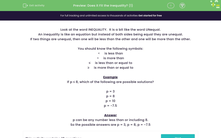# Does it Fit the Inequality

In this worksheet, students will select solutions that fit the given algebraic inequality.Key stage:  KS 3

Curriculum topic:   Algebra

Curriculum subtopic:   Understand Expressions, Equations, Inequalities, Terms and Factors

Popular topics:   Algebra worksheets

Difficulty level:#### Worksheet Overview

Look at the word inequality.  It is a bit like the word unequal.

An inequality is like an equation but instead of both sides being equal, they are unequal.

If two things are unequal, then one will be less than the other and one will be more than the other.

You should know the following symbols:

<     means less than

>     means more than

≤     means less than or equal to

≥     means more than or equal to

Example

If p + 3 ≤ 8, which of the following are possible solutions?

p = 3

p = 8

p = 10

p = -7.5

We need to find out the value of p:

p + 3 ≤ 8

Subtract 3 from both sides

p ≤ 5

So, p can be any number less than or including 5.

So the possible answers are p = 3, p = -7.5

Does that make sense?

Just as with 'normal' equations, we need to make sure that anything that we do to one side is also done to the other side - otherwise you'll get the wrong answer!!Let's have a go.

### What is EdPlace?

We're your National Curriculum aligned online education content provider helping each child succeed in English, maths and science from year 1 to GCSE. With an EdPlace account you’ll be able to track and measure progress, helping each child achieve their best. We build confidence and attainment by personalising each child’s learning at a level that suits them.

Get started